# 5 Types of Depreciation

Depreciation is the process of spreading or allocating the cost of an asset over its useful life.  Over time, the asset value will decrease due to usage, wear and tear, or obsolescence. Different companies may use different types of depreciation methods, especially those in different industries; that’s why there are many depreciation methods.

Though there are many depreciation methods, we should make sure to use the method that the depreciated value of assets over time reflects the economic benefits that the company receives from the assets. This is to align with the matching principle of accounting.

The five types of depreciation method that are commonly seen include:

1. Straight-line
2. Units of production
3. Sum of the years’ digits
4. Declining balance
5. Double-declining balance

## Straight-Line Depreciation Method

Straight-line depreciation method is the depreciation method that spread the cost of assets evenly over the useful life of the assets.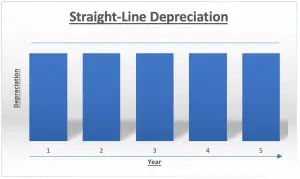The depreciation expense of the fixed assets each year, from the first year to the last year of the fixed assets, will be the same.

Straight-line depreciation is the simplest depreciation method and commonly used by the company. Hence, among all types of depreciation methods, straight-line is considered the most widely used depreciation method.

### Formula of Straight-Line Depreciation### Example of Straight-Line Depreciation

The company ABC bought a delivery truck for \$45,000 to use in the company. The company plans to use the truck for 5 years and expects to sell the truck for \$1,000 after 5 years.

What is the depreciation expense of each year using straight-line depreciation method?

Solution:

The depreciation expense of each year are:

Year 1               (45,000 – 1000)/5 = 8,800

Year 2               (45,000 – 1000)/5 = 8,800

Year 3               (45,000 – 1000)/5 = 8,800

Year 4               (45,000 – 1000)/5 = 8,800

Year 5               (45,000 – 1000)/5 = 8,800

## Units of Production Depreciation Method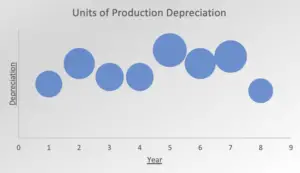Units of production depreciation is the depreciation method that uses the number of units produced as a basis for calculation. In units of production depreciation, the more that the fixed is used in the period (resulting in more units produced), the more depreciation expense will be charged.

In this method, deprecation expense is calculated by using total number of units produced in the period comparing the expected number of units that the asset produces over its useful life. Then using the rate to multiply with the asset’s net cost (cost – residual value).

Among the five types of depreciation methods, units of production depreciation may be considered the most difficult method to use as the company needs to estimate the expected number of units the asset can produce over its useful life.

### Formula of Units of Production Depreciation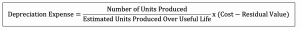### Example of Units of Production Depreciation

The company ABC bought a machine for \$40,000 for production in the company. The machine is estimated to produce 1,000 units over its useful life with a residual value of \$2,000.

In three years, the machine produced 100 units in the first year and 150 units in the second year and 130 units in the third year.

What is the depreciation expense of the first year, second and third year using units of production depreciation method?

Solution:

1st year depreciation expense = (100/1,000)*(40,000 – 2,000) = 3,800

2nd year depreciation expense = (150/1,000)*(40,000 – 2,000) = 5,700

3rd year depreciation expense = (130/1,000)*(40,000 – 2,000) = 4,940

## Sum of the Years Digits Depreciation Method

Sum of the years’ digits method is the depreciation method with the basis that the productivity of fixed asset decreases with the passage of time.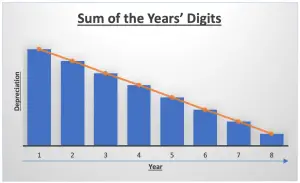It charges the depreciation amount of the fixed assets higher in the early year and the depreciation amount will keep reducing as the time passes.

In this type of depreciation, sum of the years’ digits means the summing up of each digit of depreciation year starting from the ending year of asset’s useful life to the first year. For example, if the fixed asset have useful life of 5 years, the sum of the years’s digits would equal 15 which comes from 5 + 4 + 3 + 2 + 1.

### Formula of Sum of the Years Digits Depreciation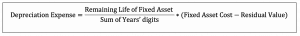### Example of Sum of the Years Digits Depreciation

The company ABC bought a machine for \$40,000 for production in the company. The machine expected to last for 8 years with the residual value of \$1,000.

What is the depreciation expense of each year using sum of the years digits depreciation method?

Solution:

The depreciation expense of each year are:

Year Sum of Years Digits Remaining Life Cost – Residual Value Depreciation Expense
1 36 8 39,000 8,667
2 36 7 39,000 7,583
3 36 6 39,000 6,500
4 36 5 39,000 5,417
5 36 4 39,000 4,333
6 36 3 39,000 3,250
7 36 2 39,000 2,167
8 36 1 39,000 1,083

*Sum of Years Digits = 1+2+3+4+5+6+7+8 = 36

## Declining Balance Depreciation Method

Declining balance depreciation is the depreciation method that reduces the net book value of the fixed assets by a fixed percentage rate.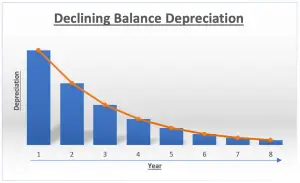This method charges the depreciation amount of fixed assets higher in the early year.

And the depreciation amount will keep reducing or declining as time passes. It does this on the basis that when a fixed asset is new it gives more benefits to the company or is more productive than when it is older.

*Net Book Value = Cost – Accumulated Depreciation

### Formula of Declining Balance Depreciation### Example of Declining Balance Depreciation

The company ABC bought a machine for \$25,000 for production in the company. The machine expected to last for 8 years with a residual value of \$800.

The management of the company estimates the machine should be depreciated at the rate of 35% annually.

What is the depreciation expense of each year using the declining balance depreciation method?

Solution:

The depreciation expense of each year are:

Year Cost Net Book Value Depreciation Rate Depreciation Expense Accumulated Depreciation
1 25,000 25,000 35% 8,750 8,750
2 25,000 16,250 35% 5,688 14,438
3 25,000 10,563 35% 3,697 18,134
4 25,000 6,866 35% 2,403 20,537
5 25,000 4,463 35% 1,562 22,099
6 25,000 2,901 35% 1,015 23,115
7 25,000 1,885 35% 660 23,774
8 25,000 1,226 35% 429 24,203

*Net Book Value after 8 years = 25,000 – 24,203 = 797

Note here that with the formula we do not need the useful life of assets to calculate depreciation; we only need depreciation rate.

In this depreciation method, the depreciation can keep going for much further than 8 years if we keep the table going with the formula. However, the depreciation usually stops when the net book value is less than or equal to residual. As in the case here, net book value after 8 years of depreciation is 797 which is less than the residual value of 800.

If there is no residual value, the depreciation will stop when the net tbook value remaining is insignificant. This also applies to the double declining depreciation method below.

## Double Declining Balance Depreciation Method

As the name suggests, double decline balance depreciation is similar to the declining balance depreciation method.It reduces the net book value of the fixed assets by a fixed percentage rate.

However, the fixed percentage rate or the depreciation rate used in this method is the double of the straight-line depreciation rate.

Double declining balance depreciation method also charges the depreciation amount of the fixed assets higher in the early year.

### Formula of Double Declining Balance Depreciation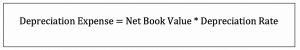### Example of Double Declining Balance Depreciation

The company ABC bought a computer for \$2,000 in which it expects to use for 4 years. The computer is expected to be sold for \$150 after 4 years.

What is the depreciation expense of each year using the double-declining balance depreciation method?

Solution:

To calculate depreciation each year using the double declining balance depreciation method here we need first determine the depreciation rate.

The straight-line depreciation rate of 4 years = 1/4 = 25% then

Double depreciation rate = 25% x 2 = 50%

The depreciation expense of each year are:

Year Cost Net Book Value Depreciation Rate Depreciation Expense Accumulated Depreciation
1 2,000 2,000 50% 1,000 1,000
2 2,000 1,000 50% 500 1,500
3 2,000 500 50% 250 1,750
4 2,000 250 50% 125 1,875

*Net Book Value after 4 years = 2,000 – 1,875 = 125